•5星
5KB m0_53867522 2021-05-25 12:53:29
•5星
18KB m0_44995828 2020-12-16 21:46:42
•5星
26KB weixin_46080777 2021-04-21 14:03:47
•5星
5.47MB weixin_44793491 2021-04-02 10:39:49
•5星
10KB lj_70596 2021-01-28 11:23:17
•5星
11.87MB weixin_44155115 2021-07-04 16:04:05
•5星
580KB weixin_40228600 2021-05-06 14:27:19
•5星
700KB qq_36686437 2021-09-03 08:34:44
•5星
27.54MB guoruibin123 2021-06-16 14:12:42
•5星
861KB trisomydroplets 2021-06-11 17:30:46
• C++map容器map查找和统计 功能描述： 对map容器进行查找数据以及统计数据 #include <iostream> #include <map> using namespace std; void test() { map<int, int>m; m.insert(pair<int, ...

C++map容器map查找和统计

功能描述：

• 对map容器进行查找数据以及统计数据
#include <iostream>
#include <map>
using namespace std;

void test()
{
map<int, int>m;
m.insert(pair<int, int>(1, 10));
m.insert(pair<int, int>(2, 20));
m.insert(pair<int, int>(3, 30));

//查找
map<int, int>::iterator pos = m.find(3);
if (pos != m.end())
{
cout << "找到了元素 key" << (*pos).first << " value =" << (*pos).second << endl;
}
else
{
cout << "未找到元素" << endl;
}

//统计
int num = m.count(3);
cout << "num = " << num << endl;
}
int main()
{
test();
return 0;
}
//查找	---find(返回的是迭代器)
//统计	--- count(对于map,结果为0或者1)

C++map容器map查找和统计

展开全文qq_36711295 2020-09-08 08:53:34
• C++ map容器用法   介绍 Map是键-值对的集合，map中的所有元素都是pair，可以使用键作为下标来获取一个值。Map中所有元素都会根据元素的值自动被排序，同时拥有实值value和键值key，pair的第一元素被视为键值，...

介绍

Map是键-值对的集合，map中的所有元素都是pair，可以使用键作为下标来获取一个值。Map中所有元素都会根据元素的值自动被排序，同时拥有实值value和键值key，pair的第一元素被视为键值，第二元素被视为实值，同时map不允许两个元素有相同的键值。

用法

头文件

#include<map>

声明及其初始化

map<key, value> m;//创建一个名为m的空map对象，其键和值的类型分别为key和value。

map<key, value> m(m2);//创建m2的副本m，m与m2必须有相同的键类型和值类型。

map<key, value> m(b,e);//创建map类型的对象m，存储迭代器b和e标记的范围内所有元素的副本，元素的类型必须能转换为pair

map<key, value, comp> mp;//comp可选，为键值对存放策略，即键的比较函数，默认标准库使用键类型定义的 < 操作符来实现键的比较。所用的比较函数必须在键类型上定义严格的弱排序，可将其理解为键类型数据上的“小于”关系。在实际应用中，键类型必须能定义 < 操作符。对于键类型，其唯一的约束就是必须支持 < 操作符。

基本操作

给map容器添加元素可通过两种方式实现：

1. 通过insert成员函数实现。
2. 通过下标操作符获取元素，然后给获取的元素赋值。 map对象的访问可通过下标和迭代器两种方式实现：
3. map的下标是键，返回的是特定键所关联的值。
4. 使用迭代器访问，iter->first指向元素的键，iter->second指向键对应的值。 使用下标访问map容器与使用下标访问vector的行为截然不同：用下标访问map中不存在的元素将导致在map容器中添加一个新的元素，这个元素的键即为该下标值，键所对应的值为空。

map容器和哈希表

对于map容器，使用其下标行为一次访问一系列的相同类型的元素，则可以将这个过程理解为构造了这些元素的一个哈希表，以统计输入单词的出现次数为例：

map<string, int> word_count;
string word;
while(cin>>word)
++word_count[word];  // 相当于生成了一个哈希表word_count

在单词的第一次出现时，会在word_count中创建并插入一个以该单词为索引的新元素，同时将它的值初始化为0。然后其值立即加1，所以每次在map中添加新元素时，所统计的次数正好从1开始。需要注意的是，使用map创建的哈希表已经按键值进行了排序，所以序列的顺序已经不再是原始的输入顺序了。

map成员函数

不修改map对象的查询操作:

m.count(k);  // 返回m中键值等于k的元素的个数。
m.find(k);  // 如果m中存在按k索引的元素，则返回指向该元素的迭代器。如果不存在，则返回结束游标end()。

对于map对象，由于map中不存在相同的两个或者多个键，所以count成员的返回值只能是0或者1，用于检查map对象中某键是否存在。find成员返回的是指向元素的迭代器，如果元素不存在，则返回end迭代器，用于读取元素而又不插入元素。

从map对象中删除元素

m.erase(k);  // 删除m中键为k的元素，返回size_type类型的值，表示删除元素的个数。
m.erase(p);  // 从m中删除迭代器p所指向的元素，p必须指向m中确实存在的元素，而且不能等于m.end()，返回void类型。
m.erase(iterator first, iterator last);  // 删除一个范围，返回void类型。

map容器提供的insert操作

m.insert(e) ;

e是一个用在m上的value_type类型的值。如果键e.first不在m中，则插入一个值为e.second的新元素；如果该键在m中已存在，那么不进行任何操作。该函数返回一个pair类型对象，包含指向键为e.first的元素的map迭代器，以及一个bool类型的对象，表示是否插入了该元素。

m.insert(beg, end);

beg和end是标记元素范围的迭代器，对于该范围内的所有元素，如果它的键在m中不存在，则将该键及其关联的值插入到m。 返回void类型。

m.insert(iter, e);

e是value_type类型的值，如果e.first不在m中，则创建新元素，并以迭代器iter为起点搜索新元素存储的位置，返回一个迭代器，指向m中具有给定键的元素。 在添加新的map元素时，使用insert成员可避免使用下标操作符带来的副作用：不必要的初始化。

展开全文zhuochuyu7096 2018-09-22 20:09:01
• C++ map容器-58-map容器大小/交换/插入/删除 c++ map常用API

接着学习map容器的常见API操作，依然是大部分前面学习过的，名称相同的API的基本调用测试。本篇学习map容器大小相关API，然后学习容器交换，容器元素插入和删除，清空等操作。 1.map容器大小操作 统计map容器大小...

接着学习map容器的常见API操作，依然是大部分前面学习过的，名称相同的API的基本调用测试。本篇学习map容器大小相关API，然后学习容器交换，容器元素插入和删除，清空等操作。

1.map容器大小操作

统计map容器大小的函数原型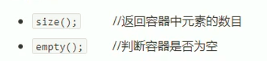#include <iostream>
#include <string>
#include <map>
using namespace std;

void test01()
{
// map容器的构造
map<string, int> m;

// map容器添加元素
m.insert(pair<string, int>("Tom", 18));
m.insert(pair<string, int>("Anthony", 23));
m.insert(pair<string, int>("Bob", 24));
m.insert(pair<string, int>("Sunny", 19));

// 统计容器大小
cout << "size of Map: " << m.size() << endl;

// 容器是否为空
cout << "is empty? " << m.empty() << endl;

}

int main()
{
test01();
system("pause");
return 0;
}

运行结果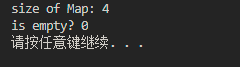数字0表示非空，数字1表示容器为空

2.map容器交换

函数原型还是swap(map)

#include <iostream>
#include <string>
#include <map>
using namespace std;

void printMap(map<string, int>& m)
{
for(map<string, int> ::iterator it = m.begin(); it != m.end(); it++)
{
cout << "Key= " << (*it).first << " ,Value= " << (*it).second << endl;
}
}

void test01()
{
// map容器的构造
map<string, int> m;

// map容器添加元素
m.insert(pair<string, int>("Tom", 18));
m.insert(pair<string, int>("Anthony", 23));
m.insert(pair<string, int>("Bob", 24));
m.insert(pair<string, int>("Sunny", 19));

map<string, int> m1;
m1.insert(pair<string, int>("aaaa", 243254));
m1.insert(pair<string, int>("bbbb", 10000));

cout << "=======m=========== " << endl;
printMap(m);
cout << "========m1========== " << endl;
printMap(m1);

cout << "================== " << endl;
// 容器交换
m.swap(m1);
cout << "=======m=========== " << endl;
printMap(m);
cout << "========m1========== " << endl;
printMap(m1);

}

int main()
{
test01();
system("pause");
return 0;
}

运行结果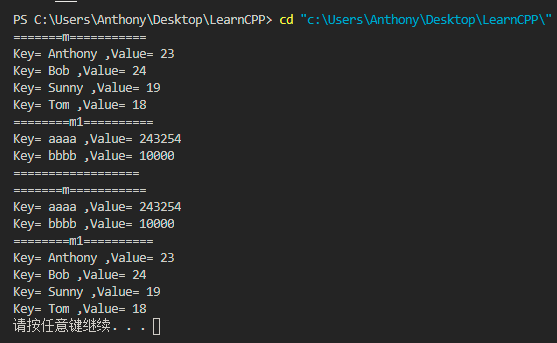3.map容器插入和删除

相关插入和删除函数原型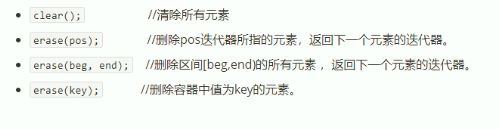插入这里不重复，就是前面一直在使用的insert()函数。

#include <iostream>
#include <string>
#include <map>
using namespace std;

void printMap(map<string, int>& m)
{
for(map<string, int> ::iterator it = m.begin(); it != m.end(); it++)
{
cout << "Key= " << (*it).first << " ,Value= " << (*it).second << endl;
}
}

void test01()
{
// map容器的构造
map<string, int> m;

// map容器添加元素
m.insert(pair<string, int>("Tom", 18));
m.insert(pair<string, int>("Anthony", 23));
m.insert(pair<string, int>("Bob", 24));
m.insert(pair<string, int>("Sunny", 19));
printMap(m);

//删除迭代器所指的元素
map<string, int>::iterator pos = m.begin();
m.erase(pos);
cout << "after erase pos=======================" << endl;
printMap(m);

//删除容器中值为Sunny
m.erase("Sunny");
cout << "after erase key=======================" << endl;
printMap(m);

// 清空容器
m.clear();
cout << "is Empty?  " <<m.empty() << endl;

}

int main()
{
test01();
system("pause");
return 0;
}

测试结果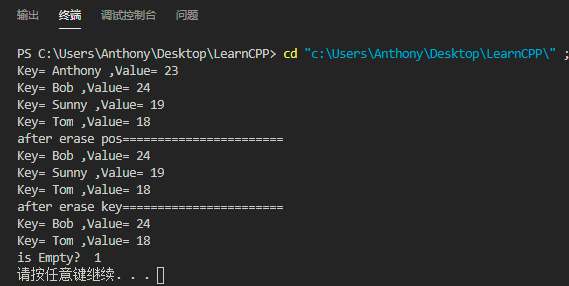4.map容器查找和统计

接着来看看查找和统计的API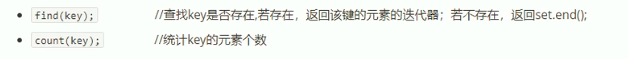#include <iostream>
#include <string>
#include <map>
using namespace std;

void printMap(map<string, int>& m)
{
for(map<string, int> ::iterator it = m.begin(); it != m.end(); it++)
{
cout << "Key= " << (*it).first << " ,Value= " << (*it).second << endl;
}
}

void test01()
{
// map容器的构造
map<string, int> m;

// map容器添加元素
m.insert(pair<string, int>("Tom", 18));
m.insert(pair<string, int>("Anthony", 23));
m.insert(pair<string, int>("Bob", 24));
m.insert(pair<string, int>("Sunny", 19));
printMap(m);

// 查找
map<string, int>::iterator pos = m.find("Anthony");
if(pos != m.end())
{
cout << "find result: " << (*pos).first << " = " << (*pos).second << endl;
}
else
{
cout << "not find"<< endl;
}

// 统计，要不是返回结果0就是1
cout << "count of Sunny："<< m.count("Sunny") << endl;

}

int main()
{
test01();
system("pause");
return 0;
}

测试结果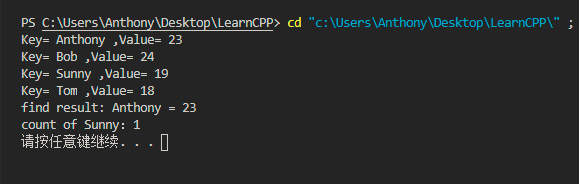展开全文u011541946 2020-08-13 23:09:29
• C++map容器-大小和互换 c++ 数据结构

统计map容器大小以及交换map容器 函数原型： 代码如下： #include <iostream> using namespace std; #include <map> //map容器 大小和交换 void test01() { map<int, int>m; m.insert(pair<...

map大小和互换
功能描述：
统计map容器大小以及交换map容器

函数原型：代码如下：

#include <iostream>
using namespace std;
#include <map>

//map容器  大小和交换
void test01() {
map<int, int>m;
m.insert(pair<int, int>(1, 10));
m.insert(pair<int, int>(2, 20));
m.insert(pair<int, int>(3, 30));

if (m.empty()) {
cout << "m为空" << endl;
} else {
cout << "m不为空" << endl;
cout << "m的大小为：" << m.size() << endl;

}
}

void printMap(map<int, int> &m) {
for (map<int, int>::iterator it = m.begin(); it != m.end(); it++) {
cout << "key = " << it->first << " " << "value = " << it->second << endl;
}
cout << endl;
}

void test02() {
map<int, int>m1;
m1.insert(pair<int, int>(1, 10));
m1.insert(pair<int, int>(2, 20));
m1.insert(pair<int, int>(3, 30));
map<int, int>m2;
m2.insert(pair<int, int>(4, 100));
m2.insert(pair<int, int>(5, 200));
m2.insert(pair<int, int>(6, 300));
cout << "交换前：" << endl;
printMap(m1);
printMap(m2);
cout << "交换后：" << endl;
m1.swap(m2);
printMap(m1);
printMap(m2);

}

int main() {

test01();
cout << "----------------------" << endl;
test02();

return 0;
}

展开全文m0_51955470 2021-01-31 00:10:06
• C++ map容器-57-对组和map构造/赋值 c++ map pair

u011541946 2020-08-12 22:42:33
• C++map容器,优势：查找 C++map容器

qq_43312665 2019-03-20 16:55:50
• 64KB weixin_38742954 2021-01-20 06:35:30
• C++ Map容器初始化遇到的问题 c++

qq_42795064 2020-05-20 16:03:57
• C++map容器基本操作大全（定义插入删除遍历清空查找排序） c++

weixin_45758146 2019-12-04 16:50:20
• C++ map容器和multimap容器（STL map容器） C map multimap

qq_28584889 2018-11-08 22:30:05
• Reborn_inuyasha 2020-06-19 17:01:02
• C++map容器循环的两种方式 C++11 map容器 auto 关联容器

qq_33237477 2018-04-13 20:21:38
• C++ map容器按value值排序 STL map

weixin_41168353 2018-07-25 15:14:26
• C++ map容器插入具有相同键的键值对的覆盖问题 c++

zmhzmhzm 2020-09-14 21:05:06
• qq_16334327 2018-07-15 12:27:44
• yanzhenyu2019 2019-11-05 16:38:43
• C++ map容器-59-map容器排序和仿函数 c++ map容器排序 函数对象 仿函数

u011541946 2020-08-16 21:56:20
• C++ map 容器 map容器4星
303KB yhs_code 2010-11-12 23:26:33
• c++ map容器的元素插入 地图

qq_31457873 2015-10-05 21:39:05
• qq_26399665 2016-10-30 00:57:34
• qq_36711295 2020-09-08 08:53:24
• 关于C++ map容器的使用和pair类型及函数 map

yo_bc 2017-03-10 11:55:13
• c++map容器介绍 c++ iterator string 存储 pair

chao_xun 2012-10-03 10:32:19
• c++ map容器 赋值及构造 判断大小 及长度详解 代码示例 数据结构 c++ 算法

qq_46721526 2020-10-18 14:02:20
• C++ map容器 c++

qq_41342326 2020-06-29 22:03:45
• qq_36711295 2020-09-08 08:54:20
• c++ map嵌套vector容器的访问 容器 c++ 运维 stl

qq_45738365 2021-12-08 21:02:32...

c++map容器c++ 订阅